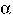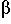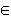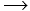LR Parsing

# LR Parsing

1. We have discussed how a shift-reduce parser works, now it is time to learn how to build one.

By way of review:

• As each input symbol is read, a shift-reduce parser either:
• pushes the symbol onto a stack which represents a prefix of the sentential form the parser believes it is parsing, or
• Pops the handle off of the top of the stack replacing all the symbols popped by the non-terminal on the left hand side of the rule used to perform the reduction.

2. In order to know when to shift and when to reduce, a bottom up parser must be able to determine when it has the handle of a sentential form sitting on top of its stack.

simple phrase
Given a grammar G and a string w =such that

1. w,,,(Vn U Vt)* ,

2. w =3. for some UVn, UP andUis a sentential form of G
we say thatis a simple phrase of the sentential form w.

handle
The leftmost simple phrase of a sentential form is called the handle.

3. One possible approach to this task is to try to make sure that the contents of the stack are always some prefix of a sentential form that may include but does not extend past the handle. We will call such a prefix a viable prefix.

4. Given that a shift-reduce parser should eventually find a rightmost derivation for any valid input, we can restrict our attention to handles of sentential forms that are encountered in rightmost derivations.

5. To get a concrete sense of what such prefixes would look like, consider the following grammar:

< E >< E > + < T >  |  < T >
< T >a  |  ( < E > )

and sample rightmost derivation in which we have displayed the handle of each sentential form in italics:

 < E >< E > + < T >< E > + a< E > + < T > + a< E > + ( < E > ) + a< E > + ( < E > + < T > ) + a< E > + ( < E > + a ) + a . . .

Any prefix of any sentential from in such a derivation that does not extend past the handle should be considered a viable prefix.

1. From the first step we would identify the following strings as viable prefixes:< E >
< E > +
< E > + < T >

2. From the second step we would identify:< E >
< E > +
< E > + a

3. Note that in this step, only the last item (which includes a part of the handle) is "new". This is true in general. So, for example, from the derivation step:< E > + ( < E > + < T > ) + a

we would only need to identify the following "new" viable prefixes:

< E > + (
< E > + ( < E >
< E > + ( < E > +
< E > + ( < E > + < T >

6. We can turn these ideas into the following formal definition.
Viable prefix
Given a grammar G, we say that(Vn U Vt)* is a viable prefix of G if there exists a rightmost derivation
SN12such that=1.

7. One way to understand the intuition behind the definition of a viable prefix is that something is a viable prefix of a sentential form it it extends up to but not past the handle..

As long as the prefix of a sentential form of a shift-reduce parser is a viable prefix for the associated grammar, things are OK (i.e. we have not yet read past the handle and there is at least some possible remaining input that could form a valid sentential form and some hope of finding a rightmost parse of this sentential form).

8. It isn't clear that identifying viable prefixes is in any way simpler than the problem of parsing itself. Basically, given the definition above, one might not expect that the set (i.e. language) of viable prefixes associated with a context-free grammar is simpler than the language associated with the grammar. Luckily, it turns out that the set of viable prefixes associated with a context free grammar forms a regular language.

We will demonstrate this by explaining how to build a finite state machine that recognizes the set of viable prefixes of a context free grammar.

9. Consider the problem of parsing strings using the following grammar:

< S >a < B >  |  b < A >  |  b c
< A >b
< B >b  |  c

• In general, we can't say whether a `b' or `c' that appears in the input is a handle or not.
• After reading a b, we know that if the following character is a `b' it is the handle, but that if it is a `c' the pair `bc' forms the handle. We even know which production to use when we reduce.
• One way to explain how we know what to do after reading a `b' is that after reading a `b' we know that we are either "in between" the `b' and the < A > in the production

< S >b < A >

and therefore also possibly at the beginning of the production

< A >b

or in between the `b' and the `c' in the production

< S >b c

10. Our approach to building LR(0) parsers will be based on a notation for describing "what point in a rule we are up to". To be precise, we need the following definitions:
LR(0) item
Given a grammar G, we say that
[ N1 .2 ]
is an LR(0) item or LR(0) configuration for G if N12 is a production in G.
Configuration Set
We will refer to a set of LR(0) items as a configuration set.

For example, the configuration set:

< S >b . < A >
< S >b . c
< A >. b

describes where we might be in various productions after reading a `b' while parsing relative to the grammar discussed above.
11. Our intuition concerning how an LR(0) item describes "where we are" is made precise by the definition:
Valid item
Given a grammar G, we say that an LR(0) item, [ N1 .2 ] , is valid for( Vn U Vt )* if there is a rightmost derivation
SN12such that1 =.

12. It should be clear that there is some connection between the definitions of valid items and viable prefixes. The connections are:
• If any LR(0) item is valid for a stringthenmust be a viable prefix.
• If some stringis a viable prefix, then there must be some LR(0) item that is valid for.
13. Since a string is a viable prefix if and only if the set of LR(0) items for the string is non-empty, building a machine that keeps track of the set of valid LR(0) items as it reads input will enable us to identify viable prefixes.
• Once such a machine starts telling us there are no valid items we will know that we are no longer looking at a viable prefix we will know that we either have reached the end of the handle or hit an error.
14. Imagine what such a machine would look like for our trivial grammar:

< S >a < B >  |  b < A >  |  b c
< A >b
< B >b  |  c

• The initial state would have to correspond to all LR(0) items valid for the null string:  [ < S >. a < B > ] [ < S >. b < A > ] [ < S >. b c ]
• From this state, there should be a transition on input a to the state corresponding to the configuration set:

[ < S >a . < B > ]
[ < B >. b ]
[ < B >. c ]

• and so on ...

Computer Science 434
Department of Computer Science
Williams CollegeLR Parsing